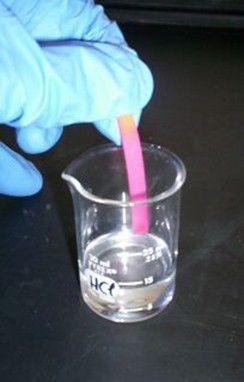## What you’ll learn to do: Rewrite logarithmic expressions using a combination of logarithm rules and formulasThe pH of hydrochloric acid is tested with litmus paper. (credit: David Berardan)

In chemistry, pH is used as a measure of the acidity or alkalinity of a substance. The pH scale runs from 0 to 14. Substances with a pH less than 7 are considered acidic and substances with a pH greater than 7 are said to be alkaline. Our bodies, for instance, must maintain a pH close to 7.35 in order for enzymes to work properly. To get a feel for what is acidic and what is alkaline, consider the following pH levels of some common substances:

• Battery acid: 0.8
• Stomach acid: 2.7
• Orange juice: 3.3
• Pure water: 7 (at 25° C)
• Human blood: 7.35
• Fresh coconut: 7.8
• Sodium hydroxide (lye): 14

To determine whether a solution is acidic or alkaline, we find its pH which is a measure of the number of active positive hydrogen ions in the solution. The pH is defined by the following formula where $a$ is the concentration of hydrogen ion in the solution.

$\begin{array}{lll}\text{pH} & = -\mathrm{log}\left(\left[{H}^{+}\right]\right)\hfill \\ \text{} & =\mathrm{log}\left(\frac{1}{\left[{H}^{+}\right]}\right)\hfill \end{array}$

$-\mathrm{log}\left(\left[{H}^{+}\right]\right)$ is equal to $\mathrm{log}\left(\frac{1}{\left[{H}^{+}\right]}\right)$ due to one of the logarithm properties we will examine in this section.

## Contribute!

Did you have an idea for improving this content? We’d love your input.